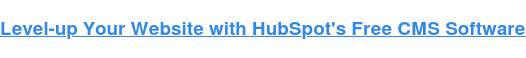# C++ String Functions: 10 Definitions + ExamplesPublished:

String functions play a key role in manipulating and processing text in C++. And, as a C++ programmer, mastering these functions is essential for completing projects and improving your overall programming skills. In this post, we'll review a comprehensive list of C++ string functions that will help you streamline your string operations and write clean and efficient code. From searching for a substring to replacing text, this list comes equipped with clear definitions and examples that will help you take your project to the next level.Without further ado, let's dive in.## C++ String Functions

### 1. String Length: std::string::length

The string length function calculates the length (number of characters) of a string.

Example:

`#include <iostream>#include <string>int main() {  std::string str = "Hello, World!";  int length = str.size();  // Returns the length of the string, which is 13  std::cout << "The length of the string is: " << length << std::endl;    return 0;}`

Output:

The length of the string is: 13

### 2. String Copy: std::strcpy

The string copy function copies a string from a source location to a destination location.

Example:

`#include <iostream>#include <cmath>int main() {    char source[] = "Hello, World!"; // Source string    char destination; // Destination character array    std::strcpy(destination, source); // Copy the source string to the destination    std::cout << "Source string: " << source << std::endl;    std::cout << "Copied string: " << destination << std::endl;    return 0;}`

Output:

Source string: Hello, World!
Copied string: Hello, World!

### 3. String Comparison: std::string::compare

The string comparison function compares two strings lexicographically and returns an integer representing the result.

Example:`#include <iostream>#include <string>int main() {  std::string str1 = "apple";  std::string str2 = "banana";  int result = str1.compare(str2);  if (result == 0) {    std::cout << "The strings are equal." << std::endl;  } else if (result < 0) {    std::cout << "The string str1 is less than str2." << std::endl;  } else {    std::cout << "The string str1 is greater than str2." << std::endl;  }  return 0;}`

Output:

The string str1 is less than str2.

### 4. String Conversion to Integer: std::stoi

This function converts a string to an integer.

Example:`#include <iostream>using namespace std;int main() {  string str = "123";  int num = stoi(str);  cout << num << endl;  return 0;}`

Output:

123

### 5. String Conversion to Double: std::stod

This function converts a string to a double.

Example:`#include <iostream>using namespace std;int main() {  string str = "3.14";  double num = stod(str);  cout << num << endl;  return 0;}`

Output:

3.14

### 6. Numeric to String Conversion: std::to_string

This function converts a number to a string.

Example:`#include <iostream>#include <string>using namespace std;int main() {  int num = 42;  string str = to_string(num);  cout << str << endl;  return 0;}`

Output:

42

### 7. String Concatenation: std::string::operator+

This function concatenates two strings.

Example:

`#include <iostream>#include <string>using namespace std;int main() {  string str1 = "Hello";  string str2 = " World!";  string result = str1 + str2;  cout << result << endl;  return 0;}`Output:

Hello World!

### 8. String Substring: std::string::substr

The string substring function extracts a substring from a string, starting at a specified position and with a specified length.

Example:

`#include <iostream>#include <string>int main() {  std::string str = "Hello, World!";  std::string substr = str.substr(7, 5);  // Extracts "World" from the original string  std::cout << "The substring is: " << substr << std::endl;  return 0;}`

Output:

The substring is: World

The string padding function pads a string with a specified character or space to a certain width.

Example:`#include <iostream>#include <string>using namespace std;int main() {  string str = "Hello";  cout << setw(10) << setfill(' ') << str << endl;  return 0;}`

Output:

Hello

### 10. String Replacement:

This function replaces a portion of a string with another string.

Example:`#include <iostream>#include <string>using namespace std;int main() {  string str = "Hello, World!";  str.replace(7, 5, "Universe");  // Replaces the substring "World" with "Universe"  cout << str << endl;=  return 0;}`

Output:

Hello, Universe!

## Using String Functions in C++

String functions are a crucial component of any programming language and C++ is no different. Once you're comfortable with these functions, you'll be able to manipulate several lines of text simultaneously to achieve whatever outcome you desire. Keep this post handy as a reference sheet that you can return to whenever you need a quick reminder on how to call string functions in C++.

Author's Note: This post was written/edited by a human with the assistance of generative AI.## Related Articles

A free suite of content management tools for marketers and developers.